Science, Maths & Technology

### Become an OU studentStart this free course now. Just create an account and sign in. Enrol and complete the course for a free statement of participation or digital badge if available.

# Exercises on Section 3

The following exercises provide extra practice on the topics covered in Section 3.

## Exercise 6 Finding quartiles and the interquartile range

(a) For the arithmetic scores in Exercise 1 (Section 1), find the quartiles and calculate the interquartile range. The stemplot of the scores is given below.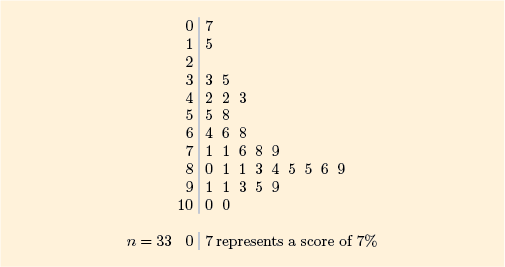Figure 30 Stemplot of arithmetic stores

### Discussion

For the arithmetic scores, so and .

The lower quartile is therefore

The upper quartile is

The interquartile range is

(b) For the television prices in Exercise 1, find the quartiles and calculate the interquartile range. The table of prices is given below.

 170 180 190 200 220 229 230 230 230 230 250 269 269 270 279 299 300 300 315 320 349 350 400 429 649 699

### Discussion

For the television prices, so and .

The lower quartile is therefore

The upper quartile is

The interquartile range is

## Exercise 7 Some five-figure summaries

Prepare a five-figure summary for each of the two batches from Exercise 1.

(a) For the arithmetic scores, the median is 79% (found in Exercise 1), and you found the quartiles and interquartile range in Exercise 6.

### Discussion

Arithmetic scores:

From the stemplot, , and .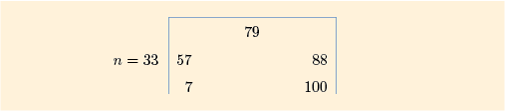Figure 31 Five-figure summary of arithmetic scores

(b) For the television prices, the median is £270 (found in Exercise 1), and you found the quartiles and interquartile range in Exercise 6.

### Discussion

Television prices:

From the data table, , and .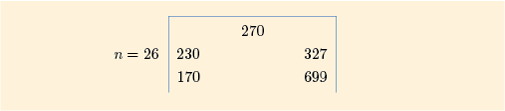Figure 32 Five-figure summary of television prices

## Exercise 8 Boxplots and the shape of distributions

Boxplots of the two batches used in Exercises 1, 6 and 7 are shown in Figures 33 and 34. On the basis of these diagrams, comment on the symmetry and/or skewness of these data.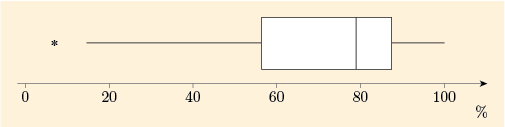Figure 33 Boxplot of batch of 33 arithmetic scores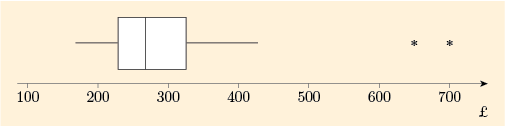Figure 34 Boxplot of batch of 26 television prices

### Discussion

For the boxplot of arithmetic scores, the left part of the box is longer than the right part, and the left whisker is also considerably longer than the right. This batch is left-skew.

For the boxplot of television prices, the right part of the box is rather longer than the left part. The right whisker is also rather longer than the left, and if one also takes into account the fact that two potential outliers have been marked, the top 25% of the data are clearly much more spread out than the bottom 25%. This batch is right-skew.